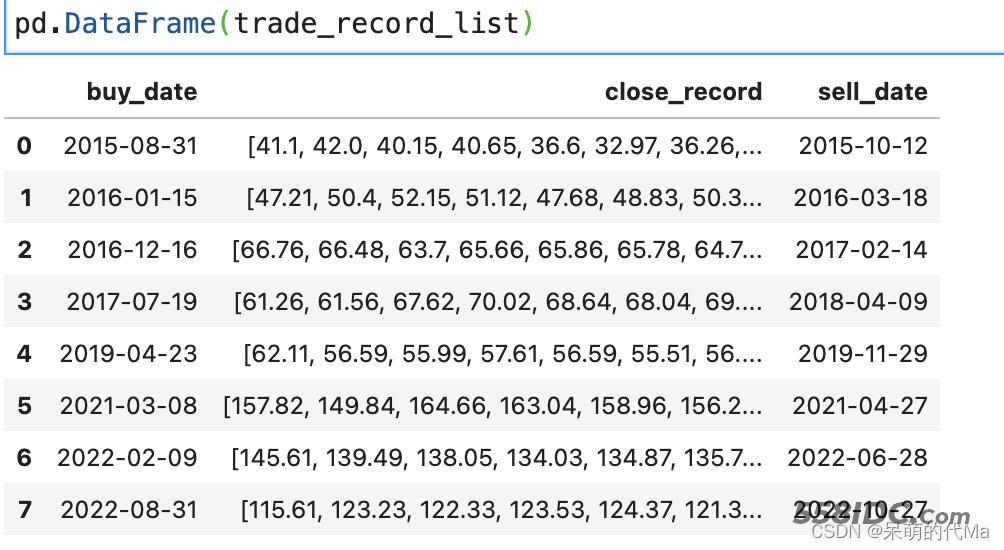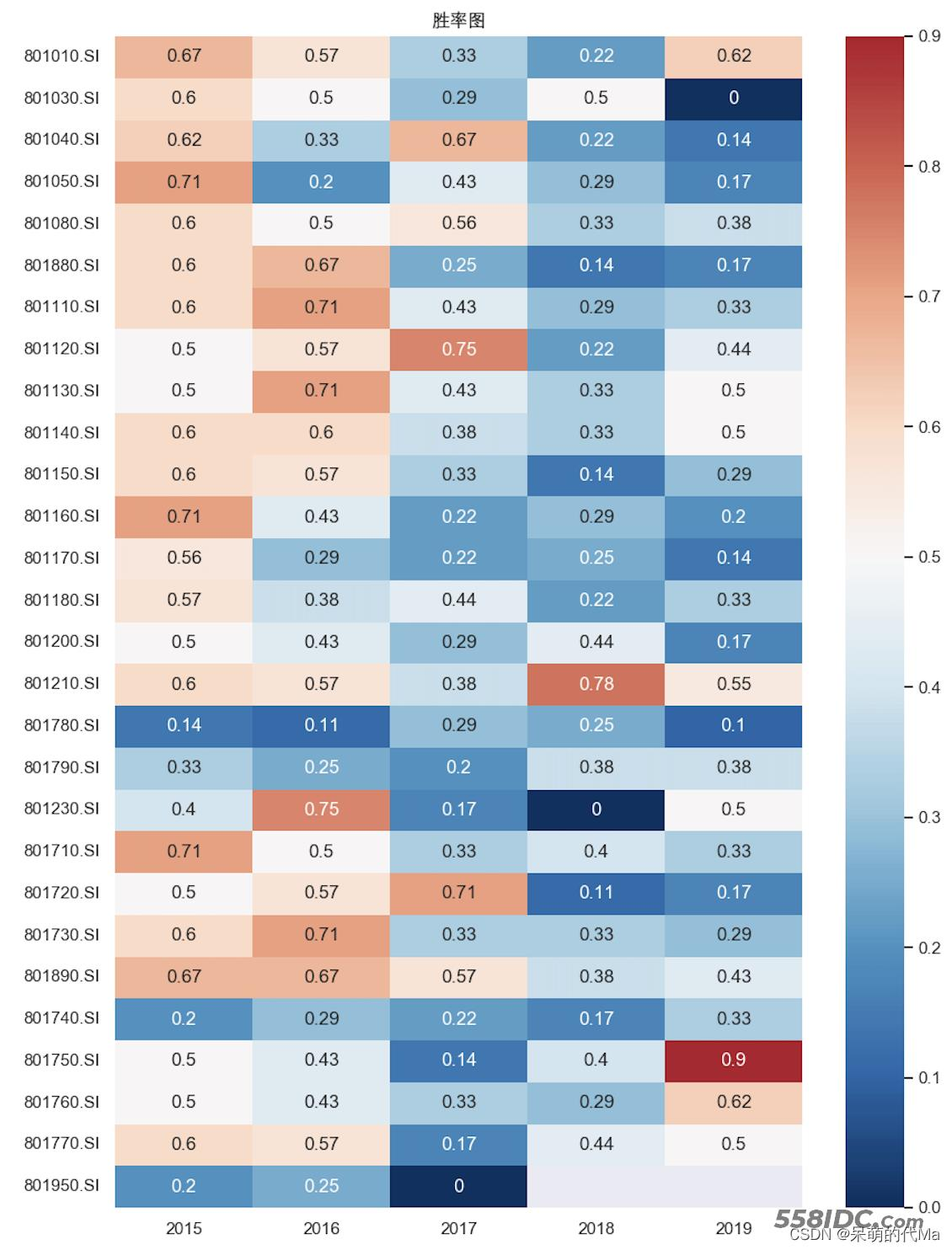﻿ Python量化因子测算与绘图超详细流程代码 - 自由资讯

# Python量化因子测算与绘图超详细流程代码

##### 目录
• 因子测算框架
• 1. 预处理股票数据
• 2. 指标测算
• 3. 测算结果整理
• 4. 结果绘图

• 测算需要用到的第三方库：numpy，pandas，talib
• 绘图需要用到的第三方库：matplotlib，seaborn

## 因子测算框架

### 1. 预处理股票数据

```# 测算用
import numpy as np
import pandas as pd
from copy import deepcopy
from tqdm import tqdm
from datetime import datetime
import talib
# 绘图用
import matplotlib as mpl
import matplotlib.pyplot as plt
import seaborn as sns
%matplotlib inline
# 绘图现实中文
sns.set()
plt.rcParams["figure.figsize"] = (20,10)
plt.rcParams['font.sans-serif'] = ['Arial Unicode MS']  # 当前字体支持中文
plt.rcParams['axes.unicode_minus'] = False  # 解决保存图像是负号'-'显示为方块的问题
# 其他
import warnings
warnings.filterwarnings("ignore")
```

```import os
files = os.listdir(path)
file_list = []
for file in files:  # 遍历文件夹
if not os.path.isdir(file):
file_list.append(path + '/' + file)
if limit:
return file_list[:limit]
return file_list
stock_dict = {}
if not _file.endswith(".pkl"):
continue
# TODO 这里可以添加筛选，是否需要将当前的股票添加到测算的股票池中
file_df.set_index(["日期"], inplace=True)
file_df.index.name = ""
file_df.index = pd.to_datetime(file_df.index)
file_df.rename(columns={'开盘':'open',"收盘":"close","最高":"high","最低":"low","成交量":"volume"},inplace=True)
stock_code = _file.split("/")[-1].replace(".pkl", '')
# TODO 这里可以添加日期，用来截取一部分数据
stock_dict[stock_code] = file_df
```

### 2. 指标测算

```for _index,_stock_df in tqdm(stock_dict.items()):
measure_df = deepcopy(_stock_df)
```

• 这里的measure_df即要测算的dataframe数据
• 使用deepcopy是防止测算的过程影响到原始数据

• 买入规则：买入信号发出&当前没有持仓，则买入
• 卖出规则：卖出信号发出&当前有持仓，则卖出
```# 开始测算
for _mea_i, _mea_series in measure_df.iterrows(): # 循环每一天
if 发出买入信号:
if this_trade is None:  # 当前没有持仓，则买入
"close_record": [_mea_series['close']],
}
elif 发出卖出信号:
if this_trade is not None:  # 要执行卖出
else:
if this_trade is not None:  # 当前有持仓
```

```{
'close_record': [41.1,42.0,40.15,40.65,36.6,32.97], # 收盘价的记录
'sell_date': Timestamp('2015-10-12 00:00:00')} # 卖出时间
# TODO 也可以添加自定义记录的指标
}
```

### 3. 测算结果整理```trade_record_df = pd.DataFrame(trade_record_list)
# TODO 这里根据自己想要的结果添加更多的测算指标
```

### 4. 结果绘图

```# 清理绘图缓存
plt.cla()
plt.clf()
# 开始绘图
plt.figure(figsize=(10, 14), dpi=100)
# 使用seaborn绘制胜率图
fig = sns.heatmap(pd.DataFrame(total_measure_record).T.round(2), annot=True, cmap="RdBu_r",center=0.5)
plt.title("胜率图")
scatter_fig = fig.get_figure()
# 保存到本地
scatter_fig.savefig("胜率图")
scatter_fig.show() # 最后显示
```﻿